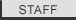DNA to Protein Tutorial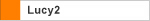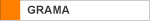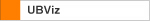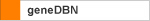Installing Perl Download Reference Manual Introduction Tutorials Numerical Data Extraction Statistical Data Extraction Patent Calculation PDB Data Extraction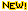GenBank Data Extraction Tabular Data AnalysisWord MappingDNA to Protein Extraction Change Log FAQ Cookbook
 Introduction This is an advanced Vect tutorial that uses just about all the rules available in Vect 1.01, so you should probably not choose this tutorial as a starting point if you are looking for an easy introduction to Vect. This tutorial uses the Arabidopsis file as an example, it can be downloaded by clicking here. For this tutorial we are going to extract protein sequences based on CDS coordinates. These coordinates will first be used to extract raw DNA sequences that go in both directions, hence some will need to be reversed. Then the DNA sequences will be converted to protein sequences and combined in the output. The above is the general idea of what we are going to do but there are many other sub-steps in this tutorial, giving a total of 18 rules to make. The final output we want is something that looks like this:Although the output looks like the extracted proteins in another simpler tutorial, it is fundamentally different. Here the proteins sequences were obtained by extracting DNA coding fragments, concatenate them, reverse complement some of them, then convert them to proteins, a substantially more complex task that demonstrates the flexibility of Vect. This tutorial has the following sections: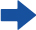Last modified June 13, 2008 . All rights reserved. Contact Webmaster